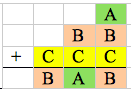#### You may also like### Consecutive Numbers

An investigation involving adding and subtracting sets of consecutive numbers. Lots to find out, lots to explore.### Calendar Capers

Choose any three by three square of dates on a calendar page...Make a set of numbers that use all the digits from 1 to 9, once and once only. Add them up. The result is divisible by 9. Add each of the digits in the new number. What is their sum? Now try some other possibilities for yourself!

# Cryptarithms

##### Age 11 to 14Challenge Level

We had lots of great solutions submitted for this problem - thank you to everybody who sent in a solution!

Here is a list of all the solutions we have managed to find for each puzzle. Please let us know if you discover any more!

1) $A=5, B=1$.

2) $A=1, B=9, C=0$.

3) $A=9, B=1, C=0$.

4) $A=9, B=2, C=1, D=0$.

5) $A=9, B=1, C=0$.

6) $A=2, B=6, C=3$
or $A=4, B=7, C=2$
or $A=6, B=8, C=1$.

7) $A=2, B=1, C=9$.

8) $A=2, B=3, C=9$.

9) $A=9, B=2, C=1$.

10) $A=5, B=0, C=1$.

11) $A=2, B=1, C=4$
or $A=2, B=6, C=5$
or $A=4, B=2, C=8$
or $A=4, B=7, C=9$.

12) $A=1, B=2, C=4$
or $A=2, B=4, C=8$
or $A=2, B=5, C=0$
or $A=3, B=7, C=4$
or $A=4, B=9, C=8$.

13) $A=5, B=9, C=6$.

14) $A=4, B=5, C=9$.

15) $A=7, B=2, C=1$.

16) $A=9, B=2, C=1, D=0, E=4$.

17) $A=1, B=8, C=5$.

18) $A=1, B=4, C=8$.

19) $A=1, B=9, C=8$.

20) $A=4, B=7, C=6$.

21) $A=2, B=9, C=8$.

22) $A=9, B=4, C=1, D=6$.

23) $A=5, B=7, 2, D=8$.

Well done to everyone who got any of these right!

Some of you gave really good explanations for how you worked out your answers.

Charlotte from St. Stephen's School in Australia explained how she got the answer to the first problem:

$A+A+A= BA$.   $A+A+A$ must equal a number above $10$ because it equals a
two digit number ($BA$). Then you must work out which three numbers under $10$
are added together to equal a number under $30$ with the same second digit. $A$
also has to be $4$ or above because $3\times3=9$ which is not a two digit number.

Then try trial and error with this information

$4+4+4= 12$
$5+5+5= 15$
$6+6+6= 18$
$7+7+7= 21$
$8+8+8= 24$
$9+9+9= 27$

By doing this you will be able to find out your answer.
$A= 5$
$B= 1$

Several people worked out that for the next few problems, the first digit of the total would be $1$. MJ from the Bourne Academy explained it like this:

I just kept thinking about how many digits were being added and found each time there was a key letter I could work out each time - $e.g.$ on question $7$, $B$ had to be $1$ as you cannot add two $2$-digit numbers together and get a number above $198$.

Lots of people used trial and error for the later problems, but some found patterns in the numbers. Here is Monique's (from St. Stephen's School) answer to question $12$:

$B$ is equal to half of $C$ and $A$ is equal to half of $B$. $A$ has to be smaller than $5$ otherwise the sum would be a $3$-digit number. $B$ can only be equal to $2$ or $4$ because they are the only numbers that can be equally divided into $A$ in the tens column.

Zach used colours to help visualise how the problems worked:

To solve the puzzles, I looked at how the letters were arranged and just thought about how numbers work.

I found that if I used colour blocks instead of letters, I could more easily visualise the relationships and very quickly arrive at the answer. Sometimes I used algebra, but generally I just looked for patterns. The questions also built understanding, and logic established in earlier questions could be assumed later on.

Here is how he used colours to help him with question $20$:"There is a number bond of $10$ in the units column, so $A+C=10$, as $B+10$ is the only feasible sum in the units column that will gie $B$ as a unit. I used trial and error to investigate number bonds of $10$, pairing $A=1$ with $C=9$ etc... $B$ then became the balancing figure. The only combination that worked was $A=4$, $B=7$, $C=6$.

Thank you again to everyone who submitted a solution!